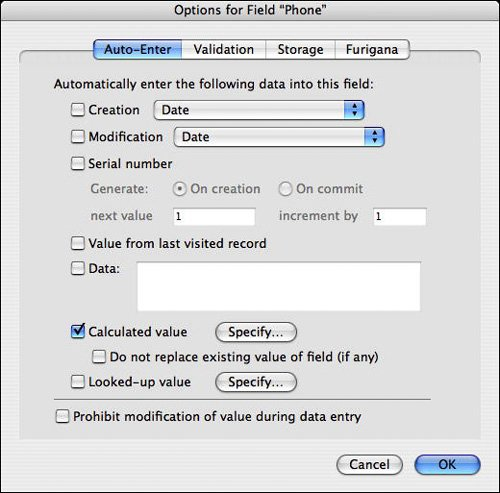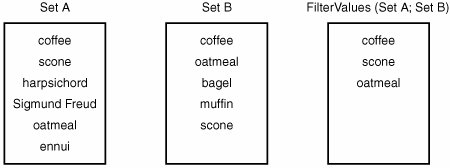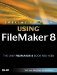# The "Filter"-ing Functions

### The Filter ing Functions

The Filter and FilterValues functions, introduced in FileMaker 7, are nifty tools for complex text comparison and manipulation. The following sections provide an example of each of them.

The Filter Function

The syntax for the Filter function is as follows:

Filter (textToFilter; filterText)

The filterText parameter consists of a set of characters that you want to "protect" in textToFilter. The output of the Filter function is the textToFilter string, minus any characters that don't appear in filterText. For example:

Filter ("This is a test" ; "aeiou") = "iiae"

Here, the filter is the set of five vowels. Therefore, the output from the function contains all the vowels from the string "This is a test". The filter is case-sensitive, so if you wanted to include both upper- and lowercase vowels in your output, you'd need to make the filterText parameter aeiouAEIOU. The output is ordered according to the order in which characters in the filter are found in the first parameter. The order of the characters in the filter itself is irrelevant.

The Filter function is useful anytime you want to constrain the domain of possible characters that a user can enter into a field. The most common use of Filter, therefore, is as part of an auto-entry calculation for text fields. Figure 14.18 shows the auto-entry options dialog for a field called Phone. Note that the option Do Not Replace Existing Value of Field (If Any) has been unchecked. What this means is that the auto-entry calculation isn't triggered only when the record is created, but also when the Phone field is modified. Essentially, this means that whenever a user modifies the Phone field, his entry is replaced immediately by the result of the calculation formula specified.

Figure 14.18. The Filter function is often used as part of the auto-entry of a calculated value.You can use the Filter function as part of the auto-entry calculation for the Phone field to remove any non-numeric characters that might have been entered by the user. The nice thing about the Filter function here is that you don't need to anticipate all the incorrect things a user can enter (text, punctuation, spaces), but rather, you can specify what the acceptable characters are. The actual function you use to reformat the user's entry in the Phone field depends on your needs and preferences, but one option would be the following:

```Let ( [
ph = Filter (Phone; "0123456789");
len = Length (ph) ;
areaCode = Case ( len = 10; Left (ph; 3); "");
exchange = Case ( len = 10; Middle (ph; 4; 3); Left (ph; 3)) ;
end = Right (ph; 4) ];

Case (
len =10 ; "(" & areaCode & ") " & exchange & " - " & end ;
len =7 ; exchange & " - " & end ;

"Error: " & TextStyleAdd ( Phone ; Bold)
)

)```

The formula starts by stripping out any non-numeric characters from the user's entry. Then, if the length of the remaining string is either 7 or 10, the number is formatted with punctuation and returned to the user. If it's not, the function shows the user an error message, complete with the original entry presented in bold text.

The FilterValues Function

The FilterValues function is similar to the Filter function, except that it filters the elements in one return-delimited set by the elements in a second return-delimited set. When each of the sets consists of unique elements, the FilterValues function essentially returns the intersection of the two sets. In Figure 14.19, you can see that FilterValues returns the items common to the two sets. Had the two parameters been reversed and the formula been written as FilterValues (Set B; Set A), the only difference would have been the order of the elements in the resulting list.

Figure 14.19. The FilterValues function returns a list of all the items of Set A that are also in Set B.Note

The result list always is ordered based on the first set. If an element appears multiple times in the first set (and it's included in the filter set), it appears multiple times in the result set.

FilterValues comes in handy anytime you want to see whether two lists contain any of the same elements. For instance, if you've defined any extended privileges as part of your security settings, you can see a list of all the privileges that have been granted to the current user with the Get (ExtendedPrivileges) function. If you have some routine that only users with PrivSetA or PrivSetC should have access to, you can use the formula FilterValues("PrivSetA¶PrivSetC"; Get (ExtendedPrivileges)). If the result is not empty, the user has at least one of those two privilege sets.

As another example, imagine that you are a third-grade teacher and that you have just given your students a 10-question True/False test. Rather than setting up a related table for their answers, you've just entered all their responses into a return-delimited text field. By also putting the answer key into a global text field, you can use the FilterValues function to determine the number of correct answers each student had. Figure 14.20 shows how this might look when you're finished. The formula for the NumberCorrect field is the following:

`ValueCount (FilterValues (TestResults; AnswerKey) )`

Figure 14.20. By using the FilterValues and ValueCount functions, you can count how many items in one array are contained within some other array.### Custom FunctionsSpecial Edition Using FileMaker 8
ISBN: 0789735121
EAN: 2147483647
Year: 2007
Pages: 296